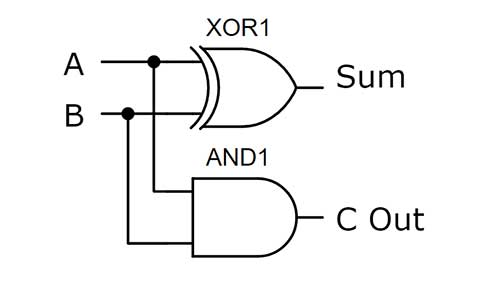A half-adder uses transistors to build logic gates which can be used to add up to the number two. Inputs A and B are the two inputs. These inputs are sent into the XOR gate and to the AND gate. The two output bits are the sum bit and the carry-out bit. This is the simplest way to build a half-adder. The XOR gate and AND gate can also be built using only NAND or NOR gates as well.The photo above shows a half adder that is built on a breadboard using NPN transistors, 2K resistors, wires, and two LEDs. Power is supplied to the breadboard from a 5-volt battery pack. The two resistors in the upper left-hand corner are inputs A and B. Both the resistors are in place so the inputs are on, the sum bit is off and the carry-out is on. The numbers being added are therefore 1 plus 1 equals 10 which is 2 in binary.

In the video above a half-adder is built and explained. Four different ways to build a full adder are also shown. A half-adder is very limited because it does not have a carry-in location. The full adder has a carry-in slot so multiple full adders can be wired together to add large numbers. While the half-adder can only add two binary numbers so the highest it can count is to the number two.The truth table makes it clear what the output should be based on the inputs. If both inputs are off both outputs are off. When one input is on the sum bit output is on making the output 01 which is one in binary. Finally when both inputs are on the carry-out and the sum bit are on making the output 10 which is two in the base 2 number system.